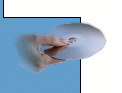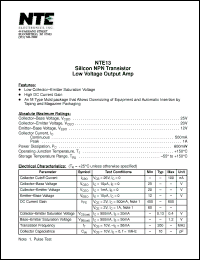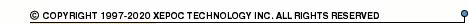More than478 969 registered clientsNTE13 series datasheets. Manufacturer: NTE.

 NTE13 Silicon NPN transistor. Low voltage output amplifier. in 3-pin -- package. Operational temperature range from 0°C to 150°C. Datasheet*) NTE130 Silicon power NPN transistor. Audio power amplifier, medium speed switch. in 3-pin TO3 package. Operational temperature range from -65°C to 200°C. Datasheet*) NTE219 Silicon power PNP transistor. Audio power amplifier, medium speed switch. in 3-pin TO3 package. Operational temperature range from -65°C to 200°C. Datasheet*) NTE1300 Integrated circuit. TV remote control. in 8-pin SIP package. Operational temperature range from -10°C to 60°C. Datasheet*) NTE1301 Integrated circuit. AM/FM IF. in 7-pin SIP package. Operational temperature range from -15°C to 60°C. Datasheet*) NTE131 Germanium complementary PNP transistor. Audio power amplifier. in 2-pin -- package. Operational temperature range from 0°C to 90°C. Datasheet*) NTE155 Germanium complementary NPN transistor. Audio power amplifier. in 2-pin -- package. Operational temperature range from 0°C to 90°C. Datasheet*) NTE1312 Integrated cicuit. Vertical OSC, amplifier. in 14-pin DIP package. Operational temperature range from -50°C to 125°C. Datasheet*) NTE1317 Integrated cicuit. Module, 2 power, 2 channel, AF power amplifier 50W Min. in 18-pin -- package. Operational temperature range from 0°C to 150°C. Datasheet*) NTE132 Silicon N-channel JFET transistor. VHF amplifier, mixer. in 3-pin -- package. Operational temperature range from -55°C to 150°C. Datasheet*) NTE1320 Integrated circuit. Module, hybrid, audio power amplifier, 25W, 2 power supplies required. in 10-pin -- package. Operational temperature range from 0°C to 150°C. Datasheet*) NTE1323 Integrated circuit. Module, hybrid, audio power amplifier, 15 Watt in 10-pin -- package. Operational temperature range from 0°C to 85°C. Datasheet*) NTE133 N-channel JFET silicon transistor. General purpose AF amplifier in 3-pin -- package. Operational temperature range from -55°C to 150°C. Datasheet*) NTE1330 Integrated circuit. Module - hybrid, dual, audio power amplifier, 15W/Ch, 2 power supplies required. in 16-pin -- package. Operational temperature range from 0°C to 105°C. Datasheet*) NTE1331 Integrated circuit. Module - dual, audio power amplifier, 25W/Channel, 2 power supplies required. in 16-pin -- package. Operational temperature range from 0°C to 105°C. Datasheet*) NTE1333 Integrated circuit. Module, hybrid, audio power amplifier, 40W, 2 power supplies required. in 10-pin -- package. Operational temperature range from 0°C to 150°C. Datasheet*) NTE1338 Integrated circuit. Module, hybrid, dual driver for 40W to 50W audio power amplifier. in 15-pin -- package. Operational temperature range from 0°C to 115°C. Datasheet*) NTE1339 Integrated circuit. Module, hybrid, dual driver for 80W to 90W audio power amplifier. in 15-pin -- package. Operational temperature range from 0°C to 115°C. Datasheet*) NTE1343 Integrated circuit. Module, hybrid, dual audio power amplifier, 10W/Ch. in 15-pin -- package. Operational temperature range from -20°C to 105°C. Datasheet*) NTE1345 Integrated circuit. Module, hybrid, dual audio power amplifier, 30W/Ch, 2 power supplies required. in 16-pin -- package. Operational temperature range from 0°C to 105°C. Datasheet*) NTE1347 Integrated circuit. Module, hybrid, dual audio power amplifier, 20W/Ch, 2 power supplies required. in 16-pin -- package. Operational temperature range from 0°C to 105°C. Datasheet*) NTE1358 Integrated circuit. Module, AF PO, 50W, dual power supply. in 10-pin -- package. Operational temperature range from 0°C to 150°C. Datasheet*) NTE1359 Integrated circuit. Module, hybrid, dual audio power amplifier, 22W/Ch. in 15-pin -- package. Operational temperature range from -20°C to 105°C. Datasheet*) NTE1365 Integrated circuit. Audio power amplifier, 5W in 9-pin SIP package. Operational temperature range from -30°C to 75°C. Datasheet*) NTE1367 Integrated circuit. Dual, audio power amplifier, 2.3W to 5W (4.6W to 15W BTL) in 18-pin DIP package. Operational temperature range from -30°C to 75°C. Datasheet*) NTE1368 Integrated circuit. Dual audio power amplifier, 5.8W/channel in 12-pin SIP package. Operational temperature range from -20°C to 75°C. Datasheet*) NTE1370 Integrated circuit. Audio power amplifier, 5.8W, for car radio. in 10-pin -- package. Operational temperature range from -20°C to 75°C. Datasheet*) NTE1375 Integrated circuit. Audio power amplifier for car radio, 5.8W. in 10-pin -- package. Operational temperature range from -20°C to 75°C. Datasheet*) NTE1376 Integrated circuit. Audio power amplifier, 22W. in 5-pin TO220 package. Operational temperature range from -40°C to 150°C. Datasheet*) NTE1377 Integrated circuit. AF power amplifier, 6W/channel. in 14-pin -- package. Operational temperature range from -20°C to 75°C. Datasheet*) NTE1378 Integrated circuit. Audio power amplifier, 10W in 5-pin TO220 package. Operational temperature range from -40°C to 150°C. Datasheet*) NTE1380 Integrated circuit. Audio power amplifier, 14W in 5-pin TO220 package. Operational temperature range from -40°C to 150°C. Datasheet*) NTE1383 Integrated circuit. Dual audio power amplifier, 5.1W/Ch (10.5W BTL) in 18-pin DIP package. Operational temperature range from -30°C to 75°C. Datasheet*) NTE1387 Integrated circuit. Dual, audio power amplifier, 2.4W/Ch. in 10-pin -- package. Operational temperature range from -20°C to 75°C. Datasheet*) NTE1388 Integrated circuit. Audio power amplifier, 5.8W, for car radio, car stereo. in 10-pin -- package. Operational temperature range from -20°C to 75°C. Datasheet*) NTE1389 Integrated circuit. Dual, audio power amplifier, 20W. in 12-pin SIP package. Operational temperature range from -30°C to 75°C. Datasheet*) NTE1390 Integrated circuit. Audio power amplifier, 12W for car radio or car stereo. in 10-pin -- package. Operational temperature range from -20°C to 75°C. Datasheet*) NTE1391 Integrated circuit. Audio power amplifier, 12W for car radio or car stereo. in 10-pin -- package. Operational temperature range from -20°C to 75°C. Datasheet*) NTE1392 Integrated circuit. Audio power amplifier, 7W. in 10-pin SIP package. Operational temperature range from -20°C to 70°C. Datasheet*) NTE1393 Integrated circuit. Audio power amplifier, 7W. in 10-pin SIP package. Operational temperature range from -20°C to 70°C. Datasheet*) NTE1394 5.5W Dual power amplifier. Car radio, car stereo output audio power amplifier. in 12-pin SIP package. Operational temperature range from -30°C to 75°C. Datasheet*) NTE1395 Integrated circuit. Dual audio power amplifier, 5.8W/Ch. in 12-pin -- package. Operational temperature range from -20°C to 70°C. Datasheet*) NTE1396 Integrated circuit. 20W bridge booster for car radio. in 11-pin -- package. Operational temperature range from -40°C to 150°C. Datasheet*) NTE1398 Integrated circuit. Dual audio power amplifier, 5.8W/Ch. in 12-pin -- package. Operational temperature range from -20°C to 70°C. Datasheet*) NTE1399 Integrated circuit. Dual audio power amplifier, 18W/Ch. in 12-pin -- package. Operational temperature range from -20°C to 70°C. Datasheet*) NTE134A Zener diode, 1 watt, +-5% tolerance. Nominal zener voltage Vz = 3.6V. Zener test current Izt = 69.0mA. in 2-pin -- package. Datasheet*) NTE135A Zener diode, 1 watt, +-5% tolerance. Nominal zener voltage Vz = 5.1V. Zener test current Izt = 49.0mA. in 2-pin -- package. Datasheet*) NTE136A Zener diode, 1 watt, +-5% tolerance. Nominal zener voltage Vz = 5.6V. Zener test current Izt = 45.0mA. in 2-pin -- package. Datasheet*) NTE137A Zener diode, 1 watt, +-5% tolerance. Nominal zener voltage Vz = 6.2V. Zener test current Izt = 41.0mA. in 2-pin -- package. Datasheet*) NTE138A Zener diode, 1 watt, +-5% tolerance. Nominal zener voltage Vz = 7.5V. Zener test current Izt = 34.0mA. in 2-pin -- package. Datasheet*) NTE139A Zener diode, 1 watt, +-5% tolerance. Nominal zener voltage Vz = 9.1V. Zener test current Izt = 28.0mA. in 2-pin -- package. Datasheet*) NTE140A Zener diode, 1 watt, +-5% tolerance. Nominal zener voltage Vz = 10.0V. Zener test current Izt = 25.0mA. in 2-pin -- package. Datasheet*) NTE141A Zener diode, 1 watt, +-5% tolerance. Nominal zener voltage Vz = 11.5V. Zener test current Izt = 22.0mA. in 2-pin -- package. Datasheet*) NTE142A Zener diode, 1 watt, +-5% tolerance. Nominal zener voltage Vz = 12.0V. Zener test current Izt = 21.0mA. in 2-pin -- package. Datasheet*) NTE143A Zener diode, 1 watt, +-5% tolerance. Nominal zener voltage Vz = 13V. Zener test current Izt = 19.0mA. in 2-pin -- package. Datasheet*) NTE144A Zener diode, 1 watt, +-5% tolerance. Nominal zener voltage Vz = 14V. Zener test current Izt = 18.0mA. in 2-pin -- package. Datasheet*) NTE145A Zener diode, 1 watt, +-5% tolerance. Nominal zener voltage Vz = 15V. Zener test current Izt = 17.0mA. in 2-pin -- package. Datasheet*) NTE146A Zener diode, 1 watt, +-5% tolerance. Nominal zener voltage Vz = 27V. Zener test current Izt = 9.5mA. in 2-pin -- package. Datasheet*) NTE147A Zener diode, 1 watt, +-5% tolerance. Nominal zener voltage Vz = 33V. Zener test current Izt = 7.5mA. in 2-pin -- package. Datasheet*) NTE148A Zener diode, 1 watt, +-5% tolerance. Nominal zener voltage Vz = 55V. Zener test current Izt = 4.75mA. in 2-pin -- package. Datasheet*) NTE149A Zener diode, 1 watt, +-5% tolerance. Nominal zener voltage Vz = 62V. Zener test current Izt = 4.0mA. in 2-pin -- package. Datasheet*) NTE150A Zener diode, 1 watt, +-5% tolerance. Nominal zener voltage Vz = 82V. Zener test current Izt = 3.0mA. in 2-pin -- package. Datasheet*) NTE151A Zener diode, 1 watt, +-5% tolerance. Nominal zener voltage Vz = 110V. Zener test current Izt = 2.3mA. in 2-pin -- package. Datasheet*) NTE5061A Zener diode, 1 watt, +-5% tolerance. Nominal zener voltage Vz = 2.4V. Zener test current Izt = 20.0mA. in 2-pin -- package. Datasheet*) NTE5062A Zener diode, 1 watt, +-5% tolerance. Nominal zener voltage Vz = 2.5V. Zener test current Izt = 20.0mA. in 2-pin -- package. Datasheet*) NTE5063A Zener diode, 1 watt, +-5% tolerance. Nominal zener voltage Vz = 2.7V. Zener test current Izt = 20.0mA. in 2-pin -- package. Datasheet*) NTE5064A Zener diode, 1 watt, +-5% tolerance. Nominal zener voltage Vz = 2.8V. Zener test current Izt = 20.0mA. in 2-pin -- package. Datasheet*) NTE5065A Zener diode, 1 watt, +-5% tolerance. Nominal zener voltage Vz = 3.0V. Zener test current Izt = 20.0mA. in 2-pin -- package. Datasheet*) NTE5066A Zener diode, 1 watt, +-5% tolerance. Nominal zener voltage Vz = 3.3V. Zener test current Izt = 76.0mA. in 2-pin -- package. Datasheet*) NTE5067A Zener diode, 1 watt, +-5% tolerance. Nominal zener voltage Vz = 3.9V. Zener test current Izt = 64.0mA. in 2-pin -- package. Datasheet*) NTE5068A Zener diode, 1 watt, +-5% tolerance. Nominal zener voltage Vz = 4.3V. Zener test current Izt = 58.0mA. in 2-pin -- package. Datasheet*) NTE5069A Zener diode, 1 watt, +-5% tolerance. Nominal zener voltage Vz = 4.7V. Zener test current Izt = 53.0mA. in 2-pin -- package. Datasheet*) NTE5070A Zener diode, 1 watt, +-5% tolerance. Nominal zener voltage Vz = 6.0V. Zener test current Izt = 43.0mA. in 2-pin -- package. Datasheet*) NTE5071A Zener diode, 1 watt, +-5% tolerance. Nominal zener voltage Vz = 6.8V. Zener test current Izt = 37.0mA. in 2-pin -- package. Datasheet*) NTE5072A Zener diode, 1 watt, +-5% tolerance. Nominal zener voltage Vz = 8.2V. Zener test current Izt = 31.0mA. in 2-pin -- package. Datasheet*) NTE5073A Zener diode, 1 watt, +-5% tolerance. Nominal zener voltage Vz = 8.7V. Zener test current Izt = 29.5mA. in 2-pin -- package. Datasheet*) NTE5074A Zener diode, 1 watt, +-5% tolerance. Nominal zener voltage Vz = 11.0V. Zener test current Izt = 23.0mA. in 2-pin -- package. Datasheet*) NTE5075A Zener diode, 1 watt, +-5% tolerance. Nominal zener voltage Vz = 16V. Zener test current Izt = 15.5mA. in 2-pin -- package. Datasheet*) NTE5076A Zener diode, 1 watt, +-5% tolerance. Nominal zener voltage Vz = 17V. Zener test current Izt = 14.75mA. in 2-pin -- package. Datasheet*) NTE5077A Zener diode, 1 watt, +-5% tolerance. Nominal zener voltage Vz = 18V. Zener test current Izt = 14.0mA. in 2-pin -- package. Datasheet*) NTE5078A Zener diode, 1 watt, +-5% tolerance. Nominal zener voltage Vz = 19V. Zener test current Izt = 13.25mA. in 2-pin -- package. Datasheet*) NTE5079A Zener diode, 1 watt, +-5% tolerance. Nominal zener voltage Vz = 20V. Zener test current Izt = 12.5mA. in 2-pin -- package. Datasheet*) NTE5080A Zener diode, 1 watt, +-5% tolerance. Nominal zener voltage Vz = 22V. Zener test current Izt = 11.5mA. in 2-pin -- package. Datasheet*) NTE5081A Zener diode, 1 watt, +-5% tolerance. Nominal zener voltage Vz = 24V. Zener test current Izt = 10.5mA. in 2-pin -- package. Datasheet*) NTE5082A Zener diode, 1 watt, +-5% tolerance. Nominal zener voltage Vz = 25V. Zener test current Izt = 10.0mA. in 2-pin -- package. Datasheet*) NTE5083A Zener diode, 1 watt, +-5% tolerance. Nominal zener voltage Vz = 28V. Zener test current Izt = 9.0mA. in 2-pin -- package. Datasheet*) NTE5084A Zener diode, 1 watt, +-5% tolerance. Nominal zener voltage Vz = 30V. Zener test current Izt = 8.5mA. in 2-pin -- package. Datasheet*) NTE5085A Zener diode, 1 watt, +-5% tolerance. Nominal zener voltage Vz = 36V. Zener test current Izt = 7.0mA. in 2-pin -- package. Datasheet*) NTE5086A Zener diode, 1 watt, +-5% tolerance. Nominal zener voltage Vz = 39V. Zener test current Izt = 6.5mA. in 2-pin -- package. Datasheet*) NTE5087A Zener diode, 1 watt, +-5% tolerance. Nominal zener voltage Vz = 43V. Zener test current Izt = 6.0mA. in 2-pin -- package. Datasheet*) NTE5088A Zener diode, 1 watt, +-5% tolerance. Nominal zener voltage Vz = 47V. Zener test current Izt = 5.5mA. in 2-pin -- package. Datasheet*) NTE5089A Zener diode, 1 watt, +-5% tolerance. Nominal zener voltage Vz = 51V. Zener test current Izt = 5.0mA. in 2-pin -- package. Datasheet*) NTE5090A Zener diode, 1 watt, +-5% tolerance. Nominal zener voltage Vz = 56V. Zener test current Izt = 4.5mA. in 2-pin -- package. Datasheet*) NTE5091A Zener diode, 1 watt, +-5% tolerance. Nominal zener voltage Vz = 60V. Zener test current Izt = 4.75mA. in 2-pin -- package. Datasheet*) NTE5092A Zener diode, 1 watt, +-5% tolerance. Nominal zener voltage Vz = 68V. Zener test current Izt = 3.7mA. in 2-pin -- package. Datasheet*) NTE5093A Zener diode, 1 watt, +-5% tolerance. Nominal zener voltage Vz = 75V. Zener test current Izt = 3.3mA. in 2-pin -- package. Datasheet*) NTE5094A Zener diode, 1 watt, +-5% tolerance. Nominal zener voltage Vz = 87V. Zener test current Izt = 2.9mA. in 2-pin -- package. Datasheet*) NTE5095A Zener diode, 1 watt, +-5% tolerance. Nominal zener voltage Vz = 91V. Zener test current Izt = 2.8mA. in 2-pin -- package. Datasheet*) NTE5096A Zener diode, 1 watt, +-5% tolerance. Nominal zener voltage Vz = 100V. Zener test current Izt = 2.5mA. in 2-pin -- package. Datasheet*) NTE5097A Zener diode, 1 watt, +-5% tolerance. Nominal zener voltage Vz = 120V. Zener test current Izt = 2.1mA. in 2-pin -- package. Datasheet*) NTE5098A Zener diode, 1 watt, +-5% tolerance. Nominal zener voltage Vz = 130V. Zener test current Izt = 1.9mA. in 2-pin -- package. Datasheet*) NTE5099A Zener diode, 1 watt, +-5% tolerance. Nominal zener voltage Vz = 140V. Zener test current Izt = 1.8mA. in 2-pin -- package. Datasheet*) NTE5100A Zener diode, 1 watt, +-5% tolerance. Nominal zener voltage Vz = 150V. Zener test current Izt = 1.7mA. in 2-pin -- package. Datasheet*) NTE5101A Zener diode, 1 watt, +-5% tolerance. Nominal zener voltage Vz = 160V. Zener test current Izt = 1.6mA. in 2-pin -- package. Datasheet*) NTE5102A Zener diode, 1 watt, +-5% tolerance. Nominal zener voltage Vz = 170V. Zener test current Izt = 1.5mA. in 2-pin -- package. Datasheet*) NTE5103A Zener diode, 1 watt, +-5% tolerance. Nominal zener voltage Vz = 180V. Zener test current Izt = 1.4mA. in 2-pin -- package. Datasheet*) NTE5104A Zener diode, 1 watt, +-5% tolerance. Nominal zener voltage Vz = 190V. Zener test current Izt = 1.3mA. in 2-pin -- package. Datasheet*) NTE5105A Zener diode, 1 watt, +-5% tolerance. Nominal zener voltage Vz = 200V. Zener test current Izt = 1.3mA. in 2-pin -- package. Datasheet*) NTE134 Zener Diode, 1 Watt ??5% Tolerance Datasheet*)